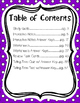# Time Math NotebookResource Type
File Type
PDF (23 MB|67 pages)
\$3.00
Product Description
*Aligns to VA SOL 3.11*

This is another one of my math notebooks, featuring time concepts, to supplement your unit. This pack includes interactive notes, worksheets to allow multiple opportunities to practice time, task cards, a study guide, quiz, and a test for the end of your unit.

What's inside:
-Study Guide
-Interactive Notes for introduction to time, how long does it take foldable, time conversions foldable, interactive clock for notebook, time to the hour, half-hour, and quarter-hour, clock sort, AM/PM, elapsed time with number line, and elapsed time by drawing clock
-Telling time to the hour and half-hour worksheet
-Telling time to the quarter-hour worksheet
-Balloon Time worksheet (time to the minute)
-Riddle worksheet (time to the minute)
-AM or PM worksheet
-Finish those Chores (elapsed time worksheet)
-Time Quiz
-End of unit test

Check out my other math notebooks!
Geometry Math Notebook
Probability Unit
Data Math Notebook
Patterns Math Notebook
Temperature Mini Unit
Place Value Math Notebook
Measurement Math Notebook

Bundle and Save on my other math notebooks!
Mega Math Notebook Growing BUNDLE
Data and Probability Notebook BUNDLE
Place Value Notebook and Word Wall BUNDLE
Measurement and Temperature Unit BUNDLE
Total Pages
67 pages